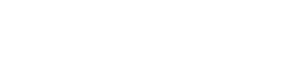Home » MPG Calculator

# MPG Calculator

Welcome to MPG calculator, our article about the calculation of miles per gallon, usually abbreviated as MPG. Our miles per gallon calculator basically divides the distance in miles by the amount of gallons used, something which is known as fuel economy. Talking about gallons, make sure to understand that the volume in a US liquid gallon (3.785411784 L) is different from the volume of an imperial gallon (4.54609 L), aka UK gallon.

0.00

Using our tool you can get both, MPG US as well as MPG UK, yet the result will be given in miles per US gallons if you choose UK gallons from the drop-down menu. Our MPG calculator is particularly useful in calculating the real MPG, which is usually less than the official or advertised fuel economy. In terms of gas cost and for the environment, the higher the MPG value the better.

Our MPG calculator is straightforward, just make sure to understand that if you choose UK gallons than the fuel economy is about twenty percent higher compared to US gallons.

To calculate MPG you have to divide the distance traveled by the gallons consumed, this gives you the true MPG. The miles per gallon formula is MPG = [mi] / [gal].

In the next section of our article you can find the frequently asked questions in the context such as what is MPG? There, we also explain the search form in the sidebar.

 👉 🛒🎁 Our TOP PICKS For You 🤩💲👍

## Miles per Gallon Calculator

You already know how to calculate miles per gallon, it’s a simple division and you can use our formula or the MPG calculator above. So, we are left with the FAQs about the topic:

• What is MPG?
• How many miles per gallon?
• How to calculate miles per gallon?
• How to figure out miles per gallon?
• How many miles to the gallon?

Taking into account all of our information, and using our miles per gallon calculator, you should have absolutely no difficulties answering these questions.

Yet, if something else is unclear send us a mail with the subject miles per gallon calculator. We use this image as summary of our article:Our frequent calculations include:

Note that instead of using our MPG calculator you can locate frequent MPG calculations using the search form in the sidebar. There, you can also look MPG conversions up.

And if you need a MPG cost calculator simply multiply the result you get using the calculator on this page with the gas price in your currency.

Submitting...